Középiskolai Matematikai és Fizikai Lapok
Informatika rovattal
 Már regisztráltál? Új vendég vagy?# Exercises and problems in Physics December 1999

## New experimental problem:

M. 211. Drops dripping from a slightly open water tap follow each other at seemingly even time intervals. Observe this phenomenon for a long period of time. How can you characterise the difference between the time intervals? (6 points)

## New problems: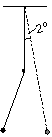P. 3294. While swinging, a thread pendulum runs into a nail each time it passes through its vertical equilibrium position. The nail is exactly at the middle of the thread. Give the difference in percentage between the time necessary for the pendulum to swing back to its original position (deviated by 2o) without and with the nail. (3 points)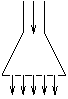P. 3295. There are 18 circular holes on a shower rose, with a diameter of 2 mm each. Water flows at a speed of 0.5 m/s through the hose with a diameter of 10 mm. At what speed does water leave the holes? Does the kinetic energy of water change? Does it increase or decrease? Give an explanation. (4 points)

P. 3296. We have N different springs. Joining them in series results in a spring with a force constant of 52 N/m. When joined in parallel, the resultant force constant is 1872 N/m. What is the maximum possible value of N? (4 points)

P. 3297. Two 1 kg lead balls start towards each other due to gravity, in an environment devoid of other gravitational effects. How long does it take them to collide if the distance between the centres of mass of the balls was initially 1 metre? (The density of lead is 11 340 kg/m3.) (4 points)

P. 3298. Using a heat engine, some air with initially a volume of 7 litres, a pressure of 105Pa and a temperature of 0 o C is driven through a thermodynamic cycle consisting of two isobar and two isochore sections. During the cycle, the air is never colder than 0 o C, and the work done by the heat engine is 500 J. What can the maximum thermodynamic efficiency of this heat engine be? (5 points)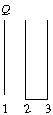P. 3299. One plate of a plane capacitor has charge Q on it, the other one is uncharged. The area of the plates is A, the distance between them is d. a) What is the voltage of the capacitor? b) What are the magnitude and the direction of the force acting on metal plate no 2 when connected with a wire to metal plate no 3 parallel to plate no 2 and with the same area as the latter (see the figure)? (4 points)

P. 3300. Can the current flowing through a consumer (e.g. the electric starter of a car) fed by an accumulator be increased by connecting a galvanic cell with the same electromotive force as the accumulator, in parallel with the latter? (3 points)

P. 3301. A one-layer solenoid is made of some metal wire with diameter d and specific resistance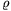by coiling the wire onto a paper cylinder of radius r, making (insulated) turns closely touching each other. The solenoid is then connected to a current supply with internal resistance R. How many turns should the solenoid have so that the energy stored in the magnetic field be maximum? (=1.78.10-8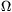m; R=1.2; d= mm; (r=3 cm. (5 points)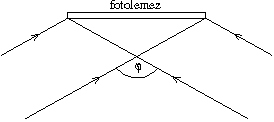P. 3302. Holographic gratings are made the following way: a parallel laser beam is divided into two beams of identical intensity that are made to interfere at angle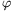at the surface of a light-sensitised plate. At what angle did the beams meet if light falling perpendicularly onto the resulting optical grating is dispersed in such a way that the complete secondary visible spectrum can just be observed? The grating was made using a He-Ne laser with wavelength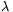0=632 nm. (The visible spectrum extends from 380 nm to 760 nm.) (5 points)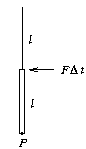P. 3303. A homogeneous rod of mass m and length l is suspended onto a thread of length l as shown in the figure. The upper end of the rod receives a small thrust F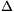t. Describe the motion of the lowest point P of the rod. (6 points)